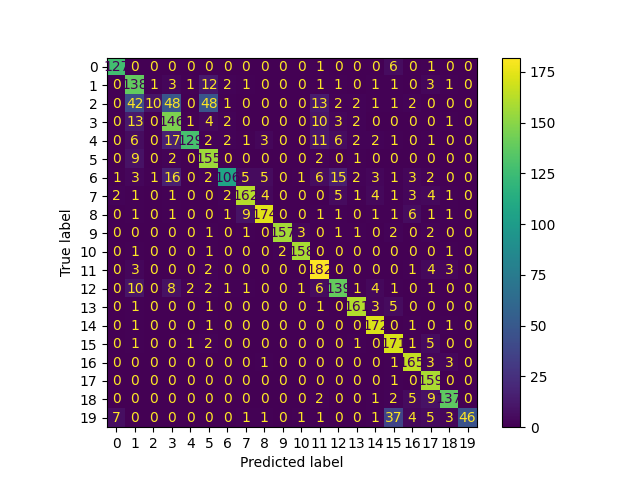# Text Classification with scikit-learn

This example shows how you can create a Hugging Face Hub compatible repo for a text classification task using scikit-learn. We also show how you can generate a model card for the model and the task at hand.

## Imports

First we will import everything required for the rest of this document.

```import pickle
from pathlib import Path
from tempfile import mkdtemp, mkstemp

import pandas as pd
import sklearn
from sklearn.datasets import fetch_20newsgroups
from sklearn.feature_extraction.text import CountVectorizer
from sklearn.metrics import (
ConfusionMatrixDisplay,
accuracy_score,
classification_report,
confusion_matrix,
f1_score,
)
from sklearn.model_selection import train_test_split
from sklearn.naive_bayes import MultinomialNB
from sklearn.pipeline import Pipeline

from skops import card, hub_utils
```

## Data

We will use 20 newsgroups dataset from sklearn. The dataset has curated news on 20 topics. It has a training and a test split.

```twenty_train = fetch_20newsgroups(subset="train", shuffle=True, random_state=42)

twenty_validation = fetch_20newsgroups(subset="test", shuffle=True, random_state=42)

X_train, X_test, y_train, y_test = train_test_split(
twenty_train.data, twenty_train.target, test_size=0.3, random_state=42
)
```

## Train a Model

To train a model, we need to convert our data first to vectors. We will use CountVectorizer in our pipeline. We will fit a Multinomial Naive Bayes model with the outputs of the vectorization.

```model = Pipeline(
[
("count", CountVectorizer()),
("clf", MultinomialNB()),
]
)

model.fit(X_train, y_train)
```
`Pipeline(steps=[('count', CountVectorizer()), ('clf', MultinomialNB())])`
In a Jupyter environment, please rerun this cell to show the HTML representation or trust the notebook.

## Inference

Let’s see if the model works.

```docs_new = [
"A graphics processing unit is a specialized electronic circuit designed to"
" manipulate and alter memory to accelerate the creation of images in a frame"
" buffer intended for output to a display device.."
]
predicted = model.predict(docs_new)
print(twenty_train.target[predicted])
```
```4
```

## Initialize a repository to save our files in

We will now initialize a repository and save our model

```_, pkl_name = mkstemp(prefix="skops-", suffix=".pkl")

with open(pkl_name, mode="bw") as f:
pickle.dump(model, file=f)

local_repo = mkdtemp(prefix="skops-")

hub_utils.init(
model=pkl_name,
requirements=[f"scikit-learn={sklearn.__version__}"],
dst=local_repo,
data=X_test,
)
```

## Create a model card

We now create a model card, and populate its metadata with information which is already provided in `config.json`, which itself is created by the call to `hub_utils.init()` above. We will see below how we can populate the model card with useful information.

```model_card = card.Card(model, metadata=card.metadata_from_config(Path(local_repo)))
```

```model_card.metadata.license = "mit"
limitations = "This model is not ready to be used in production."
model_description = (
"This is a Multinomial Naive Bayes model trained on 20 news groups dataset."
"Count vectorizer is used for vectorization."
)
model_card_authors = "skops_user"
get_started_code = (
"import pickle\nwith open(pkl_filename, 'rb') as file:\n    clf = pickle.load(file)"
)
citation_bibtex = "bibtex\n@inproceedings{...,year={2020}}"
citation_bibtex=citation_bibtex,
get_started_code=get_started_code,
model_card_authors=model_card_authors,
limitations=limitations,
model_description=model_description,
)
```
```Card(
model=Pipeline(steps=[('count', Count...rizer()), ('clf', MultinomialNB())]),
Model description/Training Procedure/Hyperparameters=TableSection(26x2),
Model description/Training Procedure/...</div></div></div></div></div></div>,
citation_bibtex=bibtex @inproceedings{...,year={2020}},
get_started_code=import pickle with o...b') as file: clf = pickle.load(file),
model_card_authors=skops_user,
limitations=This model is not ready to be used in production.,
model_description=This is a Multinomi...ectorizer is used for vectorization.,
)
```

## Add plots, metrics, and tables to our model card

We will now evaluate our model and add our findings to the model card.

```y_pred = model.predict(X_test)
eval_descr = (
"The model is evaluated on validation data from 20 news group's test split,"
" using accuracy and F1-score with micro average."
)

accuracy = accuracy_score(y_test, y_pred)
f1 = f1_score(y_test, y_pred, average="micro")

cm = confusion_matrix(y_test, y_pred, labels=model.classes_)
disp = ConfusionMatrixDisplay(confusion_matrix=cm, display_labels=model.classes_)
disp.plot()

disp.figure_.savefig(Path(local_repo) / "confusion_matrix.png")

clf_report = classification_report(
y_test, y_pred, output_dict=True, target_names=twenty_train.target_names
)
# The classification report has to be transformed into a DataFrame first to have
# the correct format. This requires removing the "accuracy", which was added
# above anyway.
del clf_report["accuracy"]
clf_report = pd.DataFrame(clf_report).T.reset_index()
folded=True,
**{
"Classification Report": clf_report,
},
)
``````Card(
model=Pipeline(steps=[('count', Count...rizer()), ('clf', MultinomialNB())]),
Model description/Training Procedure/Hyperparameters=TableSection(26x2),
Model description/Training Procedure/...</div></div></div></div></div></div>,
Model description/Evaluation Results=TableSection(2x2),
citation_bibtex=bibtex @inproceedings{...,year={2020}},
get_started_code=import pickle with o...b') as file: clf = pickle.load(file),
model_card_authors=skops_user,
limitations=This model is not ready to be used in production.,
model_description=This is a Multinomi...ectorizer is used for vectorization.,
eval_method=The model is evaluated on...acy and F1-score with micro average.,
Confusion matrix=PlotSection(confusion_matrix.png),
Classification Report=TableSection(22x5),
)
```

## Save model card

We can simply save our model card by providing a path to `Card.save()`. The model hasn’t been pushed to Hugging Face Hub yet, if you want to see how to push your models please refer to this example.

```model_card.save(Path(local_repo) / "README.md")
```

Total running time of the script: ( 0 minutes 12.316 seconds)

Gallery generated by Sphinx-Gallery